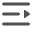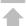# Python BSON 使用

## Python 构造 BSON 数据类型

• 整数/浮点数

Python BSON 整数/浮点数对象类型，示例数据：{"a": 123,"b":3.14}

`doc = {"a": 123,"b":3.14}`
• 字符串

Python BSON 字符串对象类型，示例数据：{"key":"hello word"}

`doc = {"key":"hello word"}`
• 列表

Python BSON 列表对象类型，示例数据：{"key":[1,2,3]}

```sublist = [1,2,3]
doc = {"key":sublist }```
• None

Python BSON None 类型，示例数据：{"key":null}，None 在数据库中会以 null 值存储。

`doc = {"key":None }`
• 对象

Python BSON 构造嵌套对象类型，示例数据：{"b":{"a":1}}

```subObj = {"a":1}
doc = {"b": subObj}```

或是

`doc = {"b":{"a":1}}`
• 对象 ID

Python BSON 构造对象 ID 类型

```# ObjectId() 可以不传入参数，这样会自动生成一个id值,推荐使用这种方式。
oid = ObjectId()
doc = {"_id" : oid}```
```#传入一个 12 字节的 str 类型参数
oid = ObjectId("5d035e2bb4d450b04fcd0dff")
doc = {"_id" : oid}```
```#传入由 24 个字符组成的 Unicode 编码参数
oid = ObjectId(u'5d035e2bb4d450b04fcd0dff')
doc = {"_id" : oid}```
• 二进制数据

Python BSON 构造二进制数类型，示例数据：{"rest": {"\$binary": "QUJD","\$type": "0"}}

```binary = Binary("ABC")
doc = {"rest" : binary}```

在构造二进制类型的数据时候也可以设置 "\$type" 的值，未设置时默认值为 0。

```subtype = 0
binary = Binary("ABC",subtype)
doc = {"rest" : binary}```
• 高精度数

Python BSON 构造不带精度要求的 Decimal 类型，示例数据： {"rest":{"\$decimal":"12345.067891234567890123456789"}}

```decimalObj = Decimal("12345.067891234567890123456789")
doc = { "rest":decimalObj }```

Python BSON 构造一个最多有 100 位有效数字，其中小数部分最多有 30 位的 Decimal 类型，示例数据： {"rest":{"\$decimal":"12345.067891234567890123456789", "\$precision":[100, 30]}}

```decimalObj =  Decimal("12345.067891234567890123456789", 100, 30)
doc = { "rest":decimalObj  }```
• 正则表达式

Python BSON 构造正则表达式数据类型，示例数据：{"regex":{"\$regex": "^w","\$options": ""}

```regexObj = Regex("^w")
doc = {"regex":regexObj }```

在构造正则表达式类型的数据时，可以设置 "\$options"。未设置时默认为 0。

```flags = 0
regexObj = Regex("^w",flags)
doc = {"regex":regexObj }```
• 日期

Python BSON 使用 datetime 的 datetime 类型来构造日期类型，示例数据：{date:{"\$date": "2019-07-12"}}

```import datetime
date = datetime.datetime(2019,07,12)
doc = {"date":date}```
• 时间戳

Python BSON 使用 Timestamp 来构造时间戳类型，示例数据：{"rest": {"\$timestamp": "2003-12-01-08.00.00.000000"}}

```time = datetime.datetime(2003,12,01,8,00,00)
timestamp = Timestamp(time,0)
doc = {"rest":timestamp}```

## 注意事项

• 特殊类型的使用注意

说明：在构建一些特殊类型的数据时（如对象 ID），如果是直接构建(如下面 condition 所示)，数据在进行 dict 转 bson 时会被认为是普通的嵌套类型（而不是对象 ID 类型）。

`condition = {"_id" : {"\$oid":"5d035e2bb4d450b04fcd0dff“}}`

以下示例用户本想构建一个对象 ID 类型作为删除匹配条件，但是由于采用了直接构建的方式，构建了一个普通嵌套类型的数据，导致匹配不到任何数据记录。

```# 错误的示例
cl = db.get_collection("sample.employee")
condition = {"_id":{"\$oid":"5d035e2bb4d450b04fcd0dff"}}
cl.delete ( condition=condition )```

正确使用示例如下，用户需要使用 ObjectId 类构建对应的对象 ID，然后再执行删除操作。

```# 正确的示例
from bson import *
oid = ObjectId("5d035e2bb4d450b04fcd0dff")
condition = {"_id":oid}
cl.delete ( condition=condition )```

其他特殊类型，如二进制数据、高精度数、日期、时间戳等，使用方式与对象 ID 类型类似，都需使用 Python 驱动的对应的构造方法来构造。收起